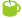### Found in titles:

1.Jython Example: stat_conf_interval.py [100%] Confidence interval for means with unknown standard deviation using the Student t distribution.
2.Jython Example: clustering_kmeans.py [100%] Run k-means cluster algorithms
3.Jython Example: clustering_tests.py [80%] Run k-means, fuzzy and hierarchical cluster algorithms
4.Jython Example: clustering_km_spmf.py [80%] Running k-means clustering over input data file
5.Jython Example: graph_medusa_kmeans.py [80%] Reading a graph from the web and running k-means clustering
6.Jython Example: jsat_kmeans.py [80%] Clustring IRIS data using k-means using JSAT
Searching 62601 articles took 5 ms